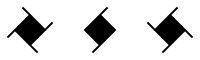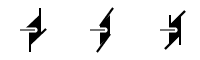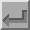Fourfold Screw Axes (41, 42, 43)The symbols above show fourfold screw axes perpendicular to the plane of the screen, i.e parallel to Z. A fourfold screw axis is equivalent to an anticlockwise rotation of 90° about a line followed by a translation parallel to that line. The translation is either 1/4, 1/2, or 3/4 of the repeat distance in the lattice along the axis for four-one, four-two, and four-three screw axes, respectively. A four-one screw axis with a fourfold rotation about the c-axis, i.e. about the line 0,0,z, followed by a translation of 1/4 c along it, will have the corresponding symmetry operator -y,x,1/4+z.

Note that four-one and four-three are equivalent except for handedness (right- and left-handed, respectively), in constrast to the four-two axis which has no handedness. These axes have the written symbols "41", "42", and "43", respectively.In space group diagrams for cubic symmetry, fourfold screw axes are shown along both X and Y directions as well as along the Z direction when present. A fractional value next to the symbol indicates the height of the axis of rotation above the XY plane.Back
 © Copyright 1995-2006.  Birkbeck College, University of London. Author(s): Jeremy Karl Cockcroft